Home
Hostname: page-component-59b7f5684b-7j4dq Total loading time: 0.21 Render date: 2022-09-28T22:27:30.458Z Has data issue: true Feature Flags: { "shouldUseShareProductTool": true, "shouldUseHypothesis": true, "isUnsiloEnabled": true, "useRatesEcommerce": false, "displayNetworkTab": true, "displayNetworkMapGraph": false, "useSa": true } hasContentIssue true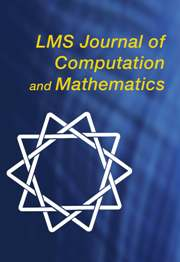LMS Journal of Computation and Mathematics

# On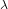${\it\lambda}$-invariants attached to cyclic cubic number fields

Published online by Cambridge University Press:  01 December 2015

## Abstract

HTML view is not available for this content. However, as you have access to this content, a full PDF is available via the ‘Save PDF’ action button.

We describe an algorithm for finding the coefficients of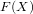$F(X)$ modulo powers of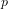$p$, where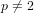$p\neq 2$ is a prime number and$F(X)$ is the power series associated to the zeta function of Kubota and Leopoldt. We next calculate the 5-adic and 7-adic${\it\lambda}$-invariants attached to those cubic extensions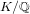$K/\mathbb{Q}$ with cyclic Galois group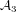${\mathcal{A}}_{3}$ (up to field discriminant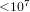${<}10^{7}$), and also tabulate the class number of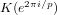$K(e^{2{\it\pi}i/p})$ for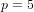$p=5$ and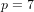$p=7$. If the${\it\lambda}$-invariant is greater than zero, we then determine all the zeros for the corresponding branches of the$p$-adic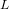$L$-function and deduce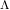${\rm\Lambda}$-monogeneity for the class group tower over the cyclotomic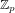$\mathbb{Z}_{p}$-extension of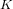$K$.

Supplementary materials are available with this article.

## MSC classification

Type
Research Article
Information
© The Author(s) 2015

## References

Childress, N. and Gold, R., ‘Zeros of p-adic L-functions’, Acta Arith. 48 (1987) 6371.Google Scholar
Delbourgo, D., ‘A Dirichlet series expansion for the p-adic zeta function’, J. Aust. Math. Soc. 81 (2006) 215224.CrossRefGoogle Scholar
Delbourgo, D., ‘The convergence of Euler products over p-adic number fields’, Proc. Edinb. Math. Soc. 52 (2009) 583606.CrossRefGoogle Scholar
Ellenberg, J., Jain, S. and Venkatesh, A., ‘Modelling 𝜆-invariants by p-adic random matrices’, Commun. Pure Appl. Math. 64 (2011) 12431262.CrossRefGoogle Scholar
Ernvall, R. and Metsänkylä, T., ‘A method for computing the Iwasawa 𝜆-invariant’, Math. Comp. 49 (1987) 281294.Google Scholar
Ernvall, R. and Metsänkylä, T., ‘Computation of the zeros of p-adic L-functions’, Math. Comp. 58 (1992) 815830.Google Scholar
Ferrero, B. and Washington, L., ‘The Iwasawa 𝜇 p -invariant vanishes for abelian number fields’, Ann. of Math. (2) 109 (1979) 377395.CrossRefGoogle Scholar
Greenberg, R., ‘A generalization of Kummer’s criterion’, Invent. Math. 21 (1973) 247254.CrossRefGoogle Scholar
Kida, M., ‘Kummer’s criterion for totally real number fields’, Tokyo J. Math. 14 (1991) 309317.CrossRefGoogle Scholar
Kubota, T. and Leopoldt, H., ‘Eine p-adische Theorie der Zetawerte, I: Einführung der p-adischen Dirichletschen L-Funktionen’, J. reine angew. Math. 214 (1964) 328339.Google Scholar
Llorente, P. and Quer, J., ‘On totally real cubic fields with discriminant D < 107 ’, Math. Comp. 50 (1988) 581594.Google Scholar
Mäki, S., The determination of units in real cyclic sextic fields , Lecture Notes in Mathematics 797 (Springer-Verlag, Berlin and New York, 1980).CrossRefGoogle Scholar
Mazur, B. and Wiles, A., ‘Class fields of abelian extensions of ℚ’, Invent. Math. 76 (1984) 179330.CrossRefGoogle Scholar
The PARI Group at Université de Bordeaux I, PARI/GP Version 2.7.0, 2014, available online from http://pari.math.u-bordeaux.fr/.Google Scholar
Roblot, X.-F., ‘Computing p-adic L-functions of totally real number fields’, Math. Comp. 84 (2015) 831874.CrossRefGoogle Scholar
Wagstaff, S. Jr., ‘Zeros of p-adic L-functions’, Math. Comp. 29 (1975) 11381143.Google Scholar
Wagstaff, S. Jr., ‘Zeros of p-adic L-functions II’, Number theory related to Fermat’s last theorem (Cambridge, Mass. 1981) , Progress in Mathematics 26 (Birkhäuser, 1982) 297308.CrossRefGoogle Scholar
Washington, L., Introduction to cyclotomic fields , 2nd edn, Graduate Texts in Mathematics 83 (Springer, 1997).CrossRefGoogle Scholar
Wiles, A., ‘The Iwasawa conjecture for totally real fields’, Ann. of Math. 131 (1990) no. 3, 493540.CrossRefGoogle Scholar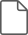### Delbourgo and Chao supplementary material

Delbourgo and Chao supplementary material 1

File 439 KBYou have Access

# Save article to Kindle

To save this article to your Kindle, first ensure coreplatform@cambridge.org is added to your Approved Personal Document E-mail List under your Personal Document Settings on the Manage Your Content and Devices page of your Amazon account. Then enter the ‘name’ part of your Kindle email address below. Find out more about saving to your Kindle.

Note you can select to save to either the @free.kindle.com or @kindle.com variations. ‘@free.kindle.com’ emails are free but can only be saved to your device when it is connected to wi-fi. ‘@kindle.com’ emails can be delivered even when you are not connected to wi-fi, but note that service fees apply.

Find out more about the Kindle Personal Document Service.

On${\it\lambda}$-invariants attached to cyclic cubic number fields Available formats × # Save article to Dropbox To save this article to your Dropbox account, please select one or more formats and confirm that you agree to abide by our usage policies. If this is the first time you used this feature, you will be asked to authorise Cambridge Core to connect with your Dropbox account. Find out more about saving content to Dropbox. On${\it\lambda}$-invariants attached to cyclic cubic number fields
Available formats
×

# Save article to Google Drive

To save this article to your Google Drive account, please select one or more formats and confirm that you agree to abide by our usage policies. If this is the first time you used this feature, you will be asked to authorise Cambridge Core to connect with your Google Drive account. Find out more about saving content to Google Drive.

On${\it\lambda}\$-invariants attached to cyclic cubic number fields
Available formats
×
×